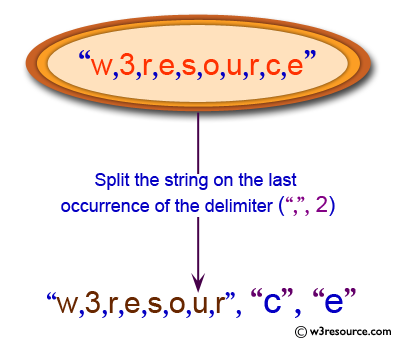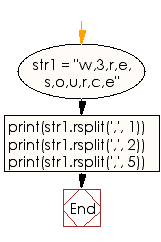﻿ Python: Split a string on the last occurrence of the delimiter - w3resource# Python: Split a string on the last occurrence of the delimiter

## Python String: Exercise-50 with Solution

Write a Python program to split a string on the last occurrence of the delimiter.Sample Solution:-

Python Code:

``````str1 = "w,3,r,e,s,o,u,r,c,e"
print(str1.rsplit(',', 1))
print(str1.rsplit(',', 2))
print(str1.rsplit(',', 5))
```
```

Sample Output:

```['w,3,r,e,s,o,u,r,c', 'e']
['w,3,r,e,s,o,u,r', 'c', 'e']
['w,3,r,e,s', 'o', 'u', 'r', 'c', 'e']
```

Flowchart:## Visualize Python code execution:

The following tool visualize what the computer is doing step-by-step as it executes the said program:

Python Code Editor:

Have another way to solve this solution? Contribute your code (and comments) through Disqus.

What is the difficulty level of this exercise?

Test your Programming skills with w3resource's quiz.

﻿

## Python: Tips of the Day

What is the difference between Python's list methods append and extend?

append: Appends object at the end.

```x = [1, 2, 3]
x.append([4, 5])
print (x)
```

Output:

```[1, 2, 3, [4, 5]]
```

extend: Extends list by appending elements from the iterable.

```x = [1, 2, 3]
x.extend([4, 5])
print (x)
```

Output:

```[1, 2, 3, 4, 5]
```

Ref: https://bit.ly/2AZ6ZFq